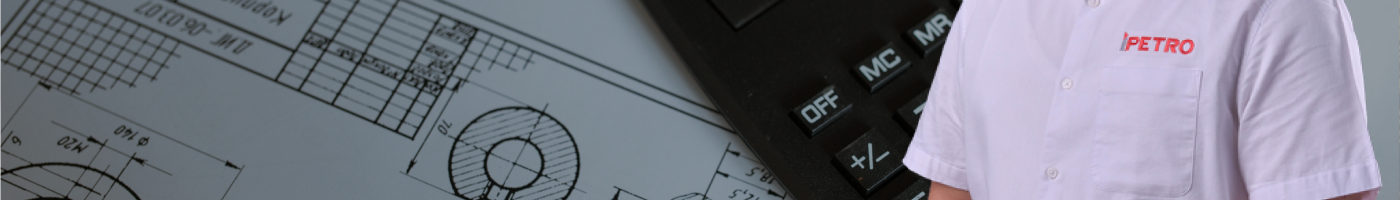# CALCULATION OF STRESSESCalculation of stresses should take into account circumferential, longitudinal, shear and equivalent stresses.

-Calculations should also take into consideration stress intensification factors that normally occur in components.

Calculation of stresses in loops, bends and offsets should be based on total range from min to max temperature normally expected.

STRESS FROM INTERNAL PRESSURE

It is calculated as:

where:

D = outside diameter of pipe, in. (mm)

Pi = internal design gage pressure, psi (bar)

SH = circumferential (hoop) stress due to internal pressure, psi (MPa)

t = wall thickness of pipe, in. (mm)

STRESS FROM EXTERNAL PRESSURE

It is calculated as:

where:

D = outside diameter of pipe, in. (mm)

Pe = external design gage pressure, psi (bar)

SH = circumferential (hoop) stress due to external pressure, psi (MPa)

t = wall thickness of pipe, in. (mm)

This code recognizes two restraint conditions:

• “Restrained” pipelines

-Sections of buried pipe.

-Above ground pipe sections attached to supports.

-Field bends in and adjacent pipe buried in stiff or consolidated soil.

• “Unrestrained” pipelines

-Above ground pipe configured to accommodate thermal expansion.

-Field bends and adjacent pipe buried in soft or unconsolidated soil.

:: ASCE Guidelines for the Design of Buried Pipelines: www.asce.org

STRESS FROM THERMAL EXPANSION

It is calculated as:

• Restrained pipe:

where:

E = Modulus of Elasticity

SE = thermal expansion stress, psi (MPE)

T1 = Temperature at installation or completion of final tie-in, 0F (0C)

T2 = operation temperature, 0F (0C)

a = coefficient of thermal expansion, in./in./0F (mm/mm/0C)

STRESS FROM THERMAL EXPANSION

It is calculated as:

• Unrestrained pipe:

where:

Sb = resultant bending stress, psi (MPa)

St = torsional stress, psi (MPa)

Sb is calculated as:

where:

ii = in-plane stress intensification factor. Note that ii, is 1 for pipe.

io = out-of-plane stress intensification factor. Note that io is 1 for pipe.

Mi = in-plane bending moment, in-Ib (Nm)

Mo = in-plane bending moment, in-Ib (Nm)

Z = Section modulus of the pipe or of the fitting outlet, in3 (cm3)

St is calculated as:

where:

Mt = torsional moment, in.-Ib (Nm)

where:

Mt = Torsional moment

Mi = In plane bending moment

Mo = Out of plane bending moment

——————————————————————————————-

Tarikh: 18 Disember 2021

Hari: Sabtu

Masa: 9.00 pagi – 4.30 petang

Platform: Zoom

Untuk mendaftar, sila klik pautan ini: https://wa.me/6001157866291?text=API+570+Webinar

## More To Explore

### ASME SECTION VIII: UG-100 PNEUMATIC TEST

Q: What is a pneumatic test? What do I need to know about it? Here are some things you need to know about pneumatic tests: